Function Repository Resource:

# GraphCount

Find the number of fundamentally different graphs of a specified size

Contributed by: Wolfram Staff (original content by Sriram V. Pemmaraju and Steven S. Skiena)
 ResourceFunction["GraphCount"][n] gives the number of nonisomorphic undirected graphs with n vertices. ResourceFunction["GraphCount"][{n,m}] gives the number of nonisomorphic undirected graphs with n vertices and m edges. ResourceFunction["GraphCount"][n,type] give the number of nonisomorphic graphs with n vertices and of the specified type. ResourceFunction["GraphCount"][{n,m},type] give the number of nonisomorphic graphs with n vertices, m edges and of the specified type.

## Details and Options

In ResourceFunction["GraphCount"][,type], type can be either "Directed" or "Undirected".
ResourceFunction["GraphCount"][n] is equivalent to ResourceFunction["GraphCount"][n,"Undirected"].

## Examples

### Basic Examples (3)

Find the number of fundamentally different (nonisomorphic) graphs with four vertices:

 In:=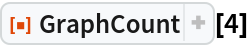Out=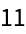Show the graphs:

 In:=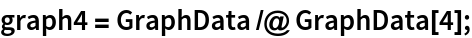In:=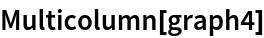Out=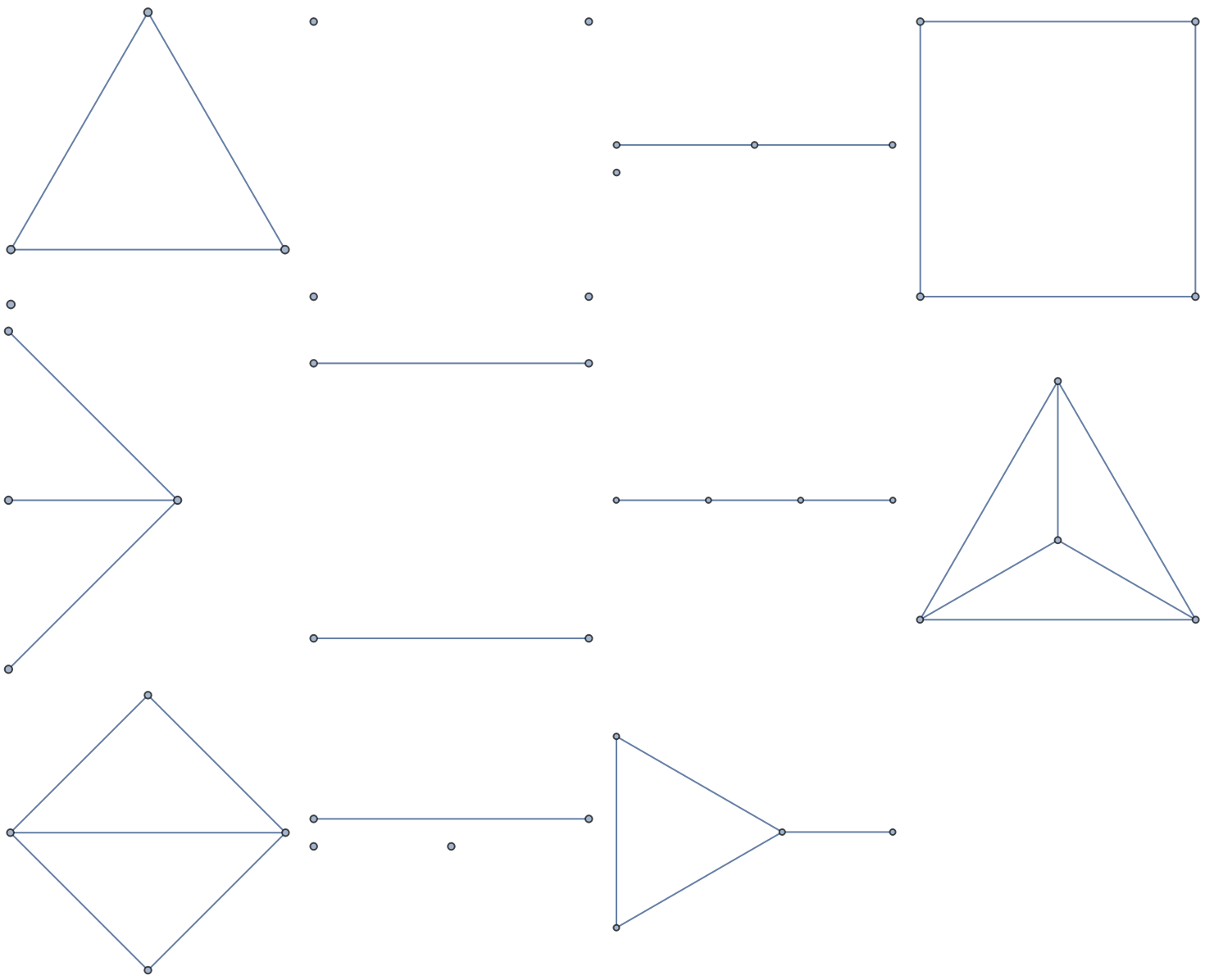In:=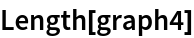Out=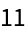Confirm that they are nonisomorphic:

 In:=Out=Find the number of graphs with four vertices and three edges:

 In:=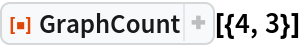Out=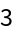Show the graphs:

 In:=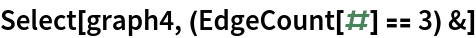Out=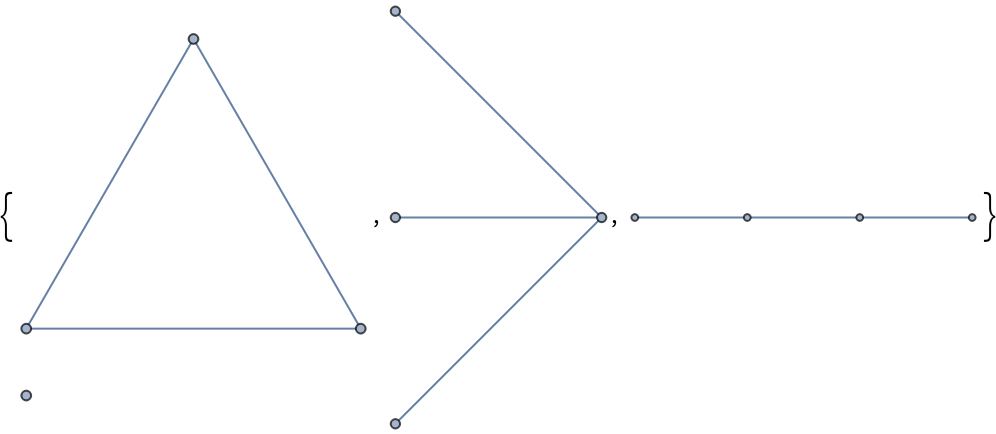In:=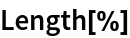Out=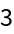The number of nonisomorphic directed graphs with three vertices:

 In:=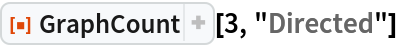Out=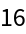### Properties and Relations (2)

The number of simple directed unlabeled graphs on n nodes for n=1,2, is given by OEIS A000273:

 In:=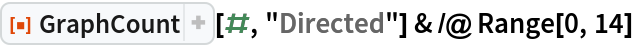Out=The number of simple directed unlabeled graphs on n nodes with m edges is given by OEIS A052283:

 In:=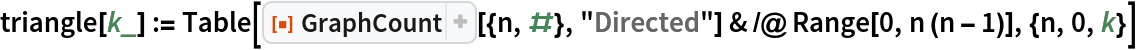In:=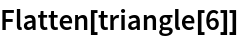Out=Or as a triangle:

 In:=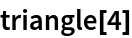Out=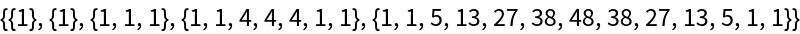In:=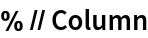Out=Sum the rows:

 In:=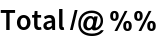Out=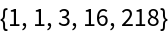This is the same as:

 In:=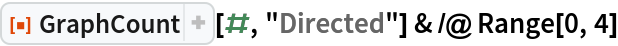Out=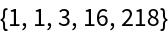### Neat Examples (1)

The number of graphs grows rapidly with the number of vertices:

 In:=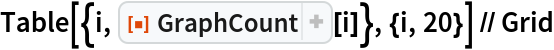Out=## Version History

• 2.0.0 – 30 September 2020
• 1.0.0 – 19 June 2020